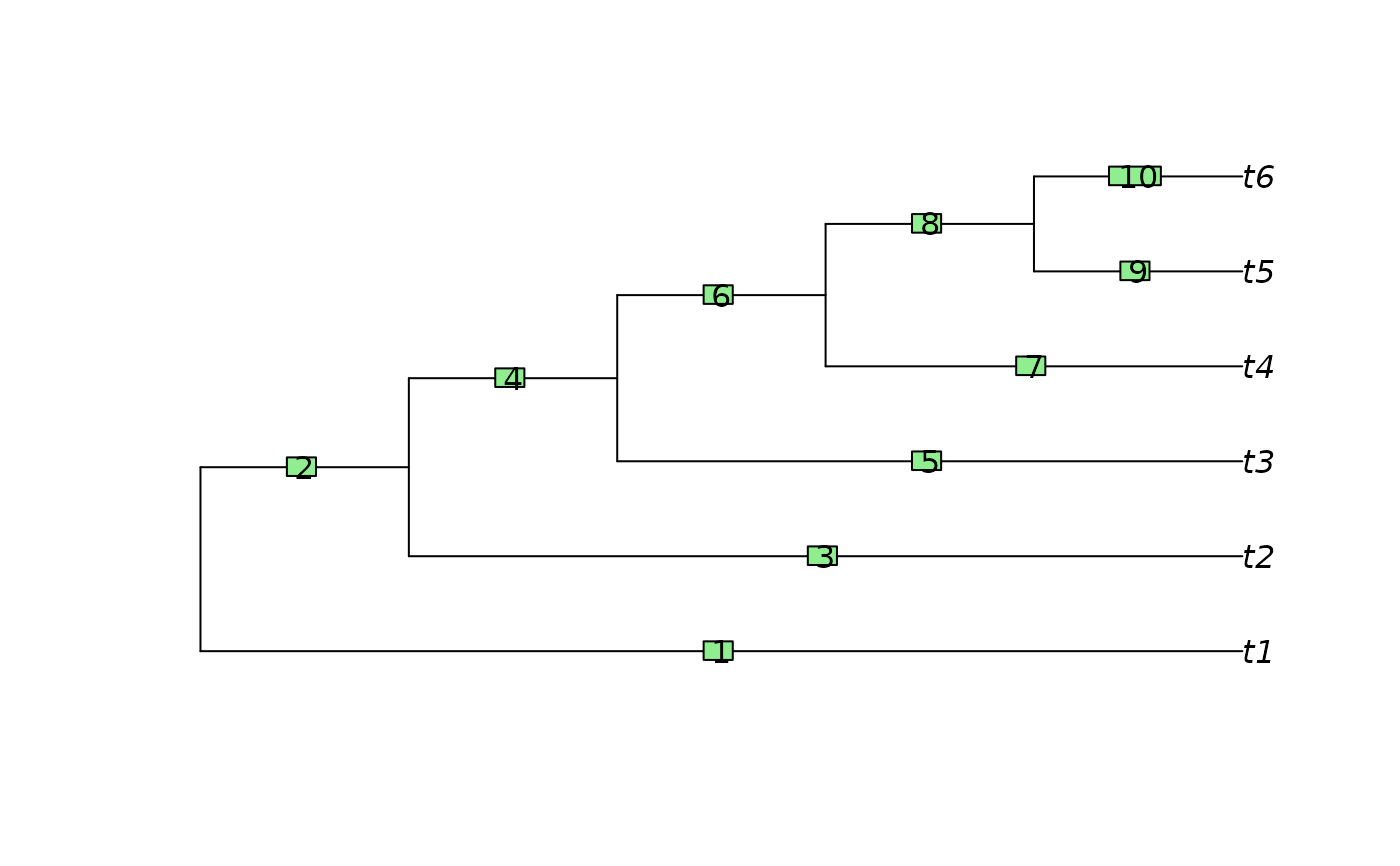Quickly identify edges that are 'ancestral' to a particular edge in a tree.

EdgeAncestry(edge, parent, child, stopAt = (parent == min(parent)))

## Arguments

edge

Integer specifying the number of the edge whose child edges should be returned.

parent

Integer vector corresponding to the first column of the edge matrix of a tree of class phylo, i.e. tree[["edge"]][, 1]

child

Integer vector corresponding to the second column of the edge matrix of a tree of class phylo, i.e. tree[["edge"]][, 2].

stopAt

Integer or logical vector specifying the edge(s) at which to terminate the search; defaults to the edges with the smallest parent, which will be the root edges if nodes are numbered Cladewise or in Preorder.

## Value

EdgeAncestry() returns a logical vector stating whether each edge in turn is a descendant of the specified edge.

Other tree navigation: AncestorEdge(), CladeSizes(), DescendantEdges(), EdgeDistances(), ListAncestors(), MRCA(), NDescendants(), NodeDepth(), NodeOrder(), NonDuplicateRoot(), RootNode()

## Author

Martin R. Smith (martin.smith@durham.ac.uk)

## Examples

tree <- PectinateTree(6)
plot(tree)
ape::edgelabels()parent <- tree$edge[, 1] child <- tree$edge[, 2]
EdgeAncestry(7, parent, child)
#>   FALSE  TRUE FALSE  TRUE FALSE  TRUE FALSE FALSE FALSE FALSE
which(EdgeAncestry(7, parent, child, stopAt = 4))
#>  4 6